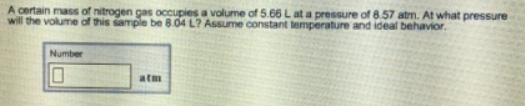# Problem: A certain mass of nitrogen gas occupies a volume of 5.66 L at a pressure of 8.57 atm. At what pressure will the volume of this sample be 8.04 L? Assume constant temperature and ideal behavior.

###### FREE Expert Solution

Boyle's Law states that as P increases, V decreases

86% (240 ratings)###### Problem Details

A certain mass of nitrogen gas occupies a volume of 5.66 L at a pressure of 8.57 atm. At what pressure will the volume of this sample be 8.04 L? Assume constant temperature and ideal behavior.